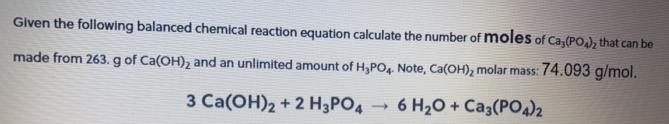# Problem: Given the following balanced chemical reaction equation calculate the number of moles of Ca3(PO4)2 that can be made from 263. g of Ca(OH)2 and an unlimited amount of H3PO4. Note, Ca(OH)2 molar mass: 74.093 g/mol. 3 Ca (OH)2 + 2H3PO4 → 6H2O + Ca3(PO4)2.

###### FREE Expert Solution
82% (123 ratings)###### Problem Details

Given the following balanced chemical reaction equation calculate the number of moles of Ca3(PO4)2 that can be made from 263. g of Ca(OH)2 and an unlimited amount of H3PO4. Note, Ca(OH)2 molar mass: 74.093 g/mol. 3 Ca (OH)2 + 2H3PO4 → 6H2O + Ca3(PO4)2